# Graphics¶

With R you can make beautiful plots. You have a lot of control over what you want your plots to look like. But all the control is via code, and this does make things pretty complicated at times.

Moreover, there are entirely different approaches to make plots. Here we only discuss scatter-plots with the “base” package. The next chapter shows other basic plot types. The chapter thereafter shows how you can make plots with additional packages `levelplot` and `ggplot`. It is very useful to learn about “base” plotting first before you get into the more complicated (and sometimes, but not always more fancy) approaches. There are many websites with cool examples.

Here we use the `cars`, `InsectSprays` and `iris` data sets that come with R.

`cars` has two variables: the speed of cars and the distances taken to stop (data recorded in the 1920s), see `?cars`

## Scatter plots¶

```data(cars)
##   speed dist
## 1     4    2
## 2     4   10
## 3     7    4
## 4     7   22
## 5     8   16
## 6     9   10
```

As we only have two variables, we can simply do

```plot(cars)
```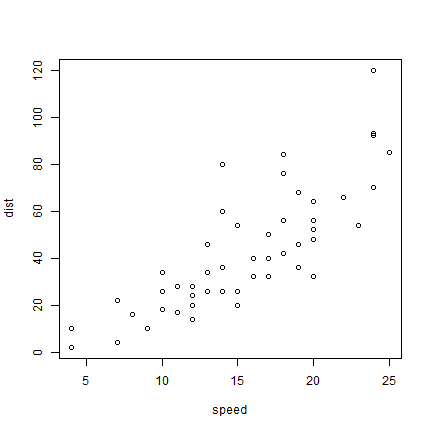But to be more explicit:

```plot(cars[,1], cars[,2])
```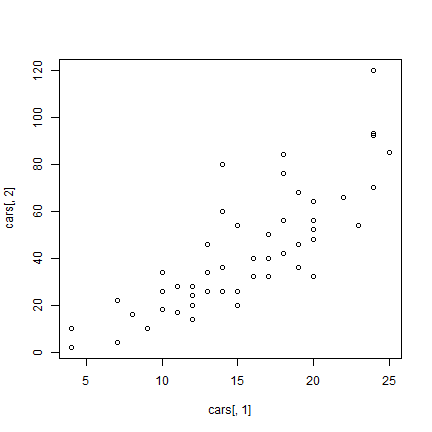And now to embellish, add axes labels.

```plot(cars[,1], cars[,2], xlab='Speed of car (miles/hr)', ylab='Stopping distance (feet)')
```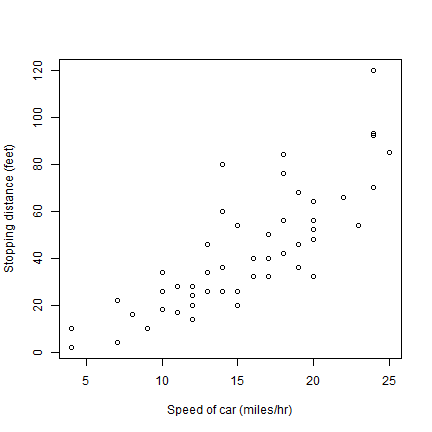Different symbols (`pch` is the symbol type, `cex` is the size).

```plot(cars, xlab='Speed of car (miles/hr)', ylab='Stopping distances (feet)', pch=20, cex=2, col='red')
```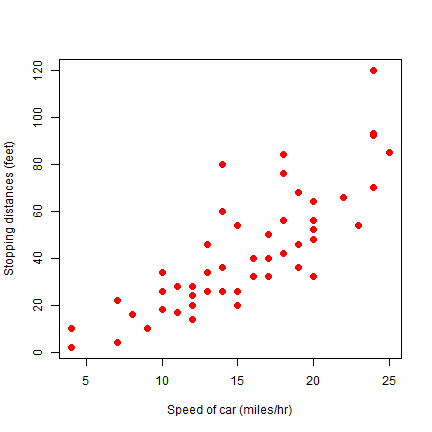`cex` is the magnification factor. The default value is 1.

Let’s change some things about the axes. Use `xlim` and `ylim` to set the start and end of an axis. `las=1` changes the orientation of the y-axis labels to horizontal.

```plot(cars, xlab='Speed', ylab='Time', pch=20, cex=2, col='red', xlim = c(0,25), las=1)
```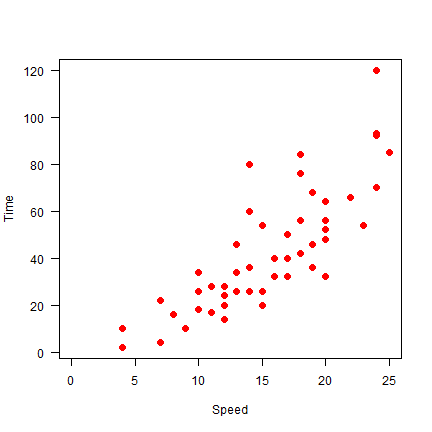Here we do not draw axes at first, and then add the lower (1) and left (2) axis, to avoid drawing the clutter from the unnecessary “upper” and “right” axis. Arguments `xaxs="i"` and `yaxs="i"` force the axis to touch at `(0,0)`.

```plot(cars, xlab='Speed', ylab='', pch=20, cex=2, col='red', xlim = c(0,27), ylim=c(0,125), axes=FALSE, xaxs="i", yaxs="i")
axis(1)
axis(2, las=1)
text(5, 100, 'Cars!', cex=2, col='blue')
par(xpd=NA)
text(-1, 133, 'Distance\n(feet)')
```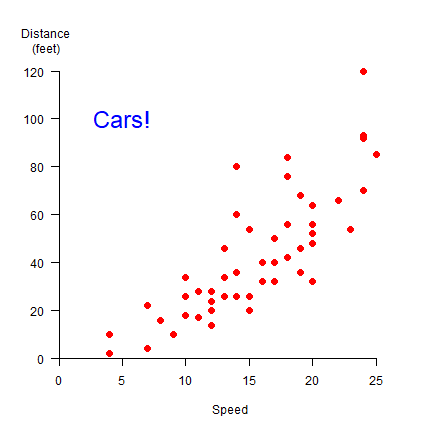We can change the symbols using another variable. Let’s say we have three car brands and that we want to vary the symbol type, color, and size by brand (typically one of these changes should suffice to distinguish them!).

```set.seed(0)
brands <- c('Buick', 'Chevrolet', 'Ford')
b <- sample(brands, nrow(cars), replace=TRUE)
i <- match(b, brands)
plot(cars, pch=i+1, cex=i, col=rainbow(3)[i])
j <- 1:length(brands)
legend(5, 120, brands, pch=(j+1), pt.cex=j, col=rainbow(3), cex=1.5)
```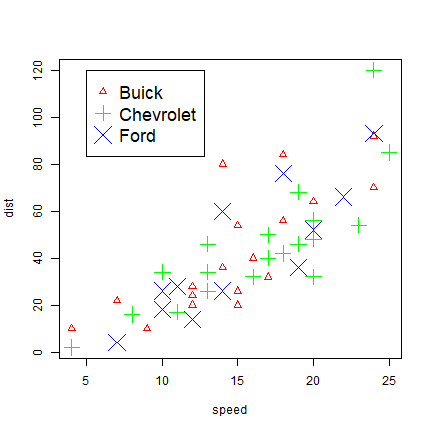The important step is the use of `match`, that creates for each character string a matching number that can be used to set the character type desired.

As you have seen above, `plot` takes many variables. Several other parameters influencing your plot, can be set with `par`. See `?par` for details. Here I use it to create 4 subplots (`mfrow=c(2,2)` with non-default margins (`mar=c(2,3,1.5,1.5)`).

```par(mfrow=c(2,2), mar=c(2,3,1.5,1.5))
for (i in 1:4) {
plot(sample(cars[,1]), sample(cars[,2]), xlab='', ylab='', las=1)
}
```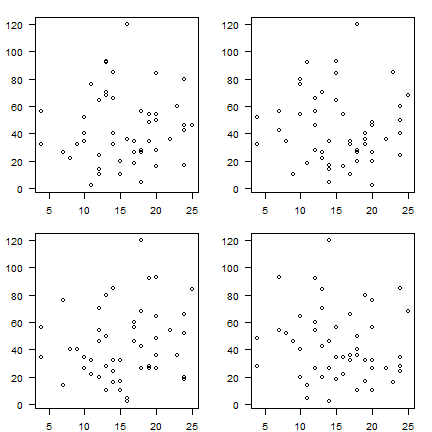## Some other base plots¶

Now consider the `InsectSprays` dataset

```head(InsectSprays)
##   count spray
## 1    10     A
## 2     7     A
## 3    20     A
## 4    14     A
## 5    14     A
## 6    12     A
```
```hist(InsectSprays[,1])
```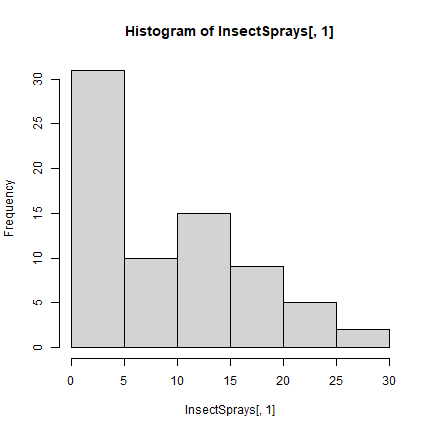```x <- aggregate(InsectSprays[,1,drop=F], InsectSprays[,2,drop=F], sum)
barplot(x[,2], names=x[,1], horiz=T, las=1)
```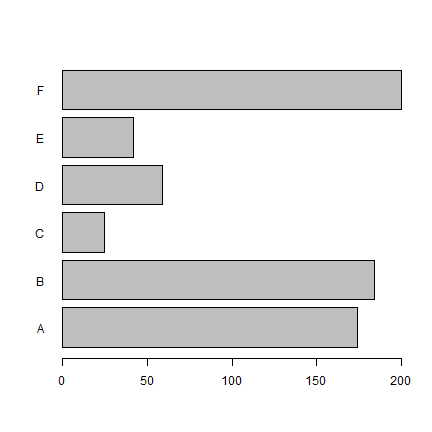```boxplot(count ~ spray, data = InsectSprays, col = "lightgray")
```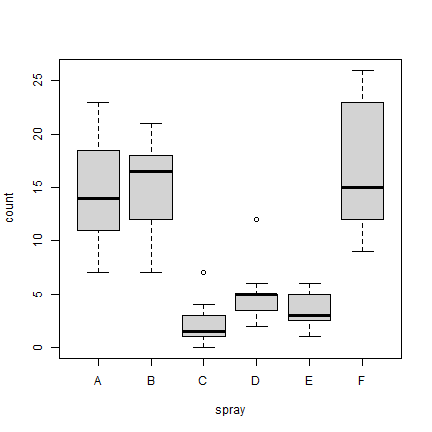## Exploring the iris data set¶

Now let’s have a look at the ‘iris’ dataset. It has 150 observations of 5 variables for 3 species of iris.

```data(iris)
str(iris)
## 'data.frame':    150 obs. of  5 variables:
##  \$ Sepal.Length: num  5.1 4.9 4.7 4.6 5 5.4 4.6 5 4.4 4.9 ...
##  \$ Sepal.Width : num  3.5 3 3.2 3.1 3.6 3.9 3.4 3.4 2.9 3.1 ...
##  \$ Petal.Length: num  1.4 1.4 1.3 1.5 1.4 1.7 1.4 1.5 1.4 1.5 ...
##  \$ Petal.Width : num  0.2 0.2 0.2 0.2 0.2 0.4 0.3 0.2 0.2 0.1 ...
##  \$ Species     : Factor w/ 3 levels "setosa","versicolor",..: 1 1 1 1 1 1 1 1 1 1 ...
```

Default plot characteristics are not satisfactory most of the time. You may want different heading, labels of x and y axis or different color of lines/points/bars. There is a large number of graphical parameters to set these. Here are some examples.

### Scatter plot¶

Note how the scatter plot type is controlled by `type` parameter.

```plot(iris\$Sepal.Length, type = 'l')
```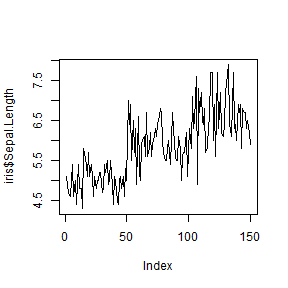```plot(iris\$Sepal.Length, type = 'p')
```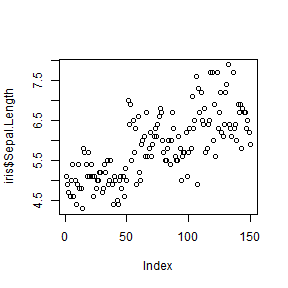```plot(iris\$Sepal.Length, type = 'o')
```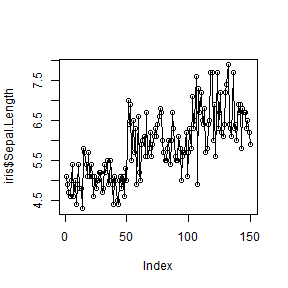```plot(iris\$Sepal.Length, iris\$Sepal.Width, type = 'p')
```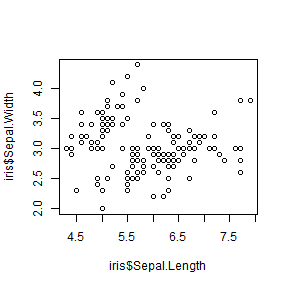### Titles and axis labels¶

```plot(iris\$Petal.Length, iris\$Petal.Width, main = "Edgar Anderson's Iris Data",
xlab = "Petal Length", ylab = "Petal Width")
```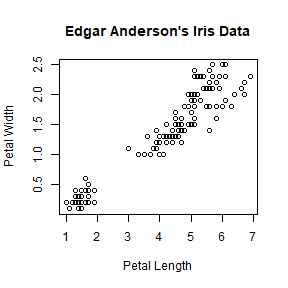The font size can be changed by `cex.main` and `cex.lab` argument. Try setting `cex.lab = 2` to increase the font size of the axes. `cex.axis` controls the size of axis tick values.

### Plotting characters¶

You can use `lty` to change the line type, and `pch` to choose a point type.

```plot(iris\$Sepal.Length, type = 'l', lty = 2, lwd = 2,
main = "Edgar Anderson's Iris Data", xlab = "Petal Length", ylab = "Petal Width")
```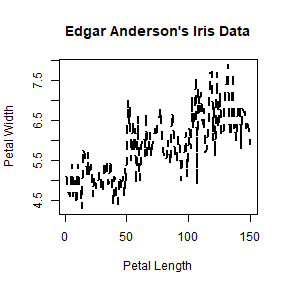```plot(iris\$Petal.Length, iris\$Petal.Width, pch = 8,
main = "Edgar Anderson's Iris Data", xlab = "Petal Length", ylab = "Petal Width")
```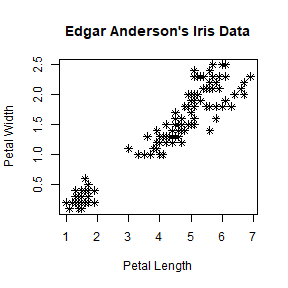There are many choices for `pch`. You can use `example("pch")`, or do `plot(1:25, pch=1:25)` to see the some plotting characters. Instead of a code, you can also specify the character like in `pch="&"`.

The size of a point character (pch) can be controlled with `cex` argument.

### Colors¶

```plot(iris\$Petal.Length, iris\$Petal.Width, pch = 8, col = 'red',
main = "Edgar Anderson's Iris Data", xlab = "Petal Length", ylab = "Petal Width")
```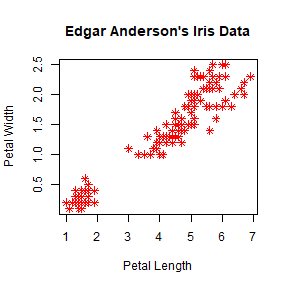We can link a color to a value.

```mycolor <- c("red","green3","blue")[as.factor(iris\$Species)]
plot(iris\$Petal.Length, iris\$Petal.Width, pch = 8, col = mycolor,
main = "Edgar Anderson's Iris Data", xlab = "Petal Length", ylab = "Petal Width")
```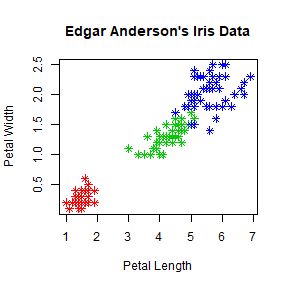Note that the length of `col` vector should be equal to the number of items you are plotting.

You can use `colors()` to see a list of named colors.

### Axes¶

To change the range of an axis you can use `xlim` and/or `ylim` argument(s).

```plot(iris\$Petal.Length, iris\$Petal.Width, pch = 8, col = mycolor,
main = "Edgar Anderson's Iris Data", xlab = "Petal Length", ylab = "Petal Width",
xlim = c(0,10), ylim= c(0,10))
```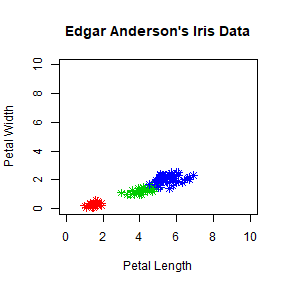You can also set `xlim` and `ylim` with `range`. Try `xlim = range(iris\$Petal.Length)`.

Use `axis` function to change the tick position and annotations (axes labels). First you need to turn off the default axes.

```plot(iris\$Petal.Length, iris\$Petal.Width, pch = 8, col = mycolor,
main = "Edgar Anderson's Iris Data", xlab = "Petal Length", ylab = "Petal Width",
xlim = c(0,10), ylim= c(0,10), axes = FALSE)
axis(1, at = 1:10, lab = c(1:10))
axis(2, at = 1:10, lab = c(1:10))
```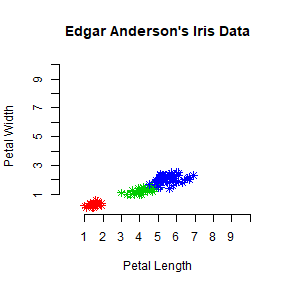### Interacting with plots¶

You can use `identify()` to identify a particular point in the plot. Try `identify(iris\$Petal.Length, iris\$Petal.Width)`. You can left click with the mouse to identify multiple points. Once complete use `ESC` to end the process.

You can use `locator()` to find out the coordinates at a particular position on a graph. Try `locator()` . You can left click with the mouse any number of times within the axes and use `ESC` to end the process. A list of X and Y coordinates of the positions clicked will be returned. You can retain this information by assigning a variable to locator before starting it: `loc <- locator()`. The coordinates will be stored as `loc\$x` and `loc\$y`. `locator` is particularly useful to add additional information to the graph. See the following example.

### Legend¶

We use a different color to represent each species of iris. We can create a legend for this information using `legend` function.

```plot(iris\$Petal.Length, iris\$Petal.Width, pch = 8, col = mycolor,
main = "Edgar Anderson's Iris Data", xlab = "Petal Length", ylab = "Petal Width",
xlim = c(0,10), ylim= c(0,10))
legend('topright', legend = unique(iris\$Species), col = c("red","green3","blue"), pch = 8)
```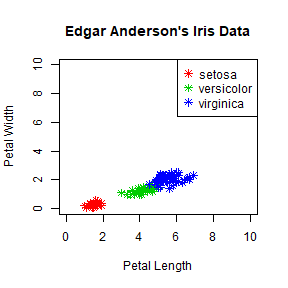To make a legend with no border use `bty = 'n'`.

Often we want include additional text in the plot. `locator` can be used to find the approximate x and y coordinates where you want to place the text. Use `loc <- locator()`.

```loc <- list()
loc\$x <- 2.75
loc\$y <- 4.94
plot(iris\$Petal.Length, iris\$Petal.Width, pch = 8, col = mycolor,
main = "Edgar Anderson's Iris Data", xlab = "Petal Length", ylab = "Petal Width",
xlim = c(0,10), ylim= c(0,10))
legend('topright', legend = unique(iris\$Species), col = c("red","green3","blue"),
pch = 8, bty = 'n')
text(loc\$x, loc\$y, labels = "Hello! this is a Scatter Plot")
```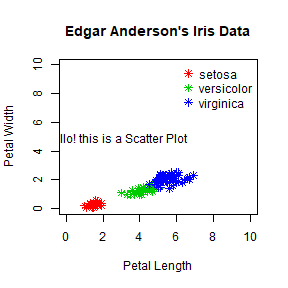## Multiple plots¶

To combine multiple plots in one image you can use `layout()` or `par()`. For example, 4 plots can be combined with `layout(matrix(1:4, 2, 2))` or `par(mfrow=c(2,2))`.

```par(mfrow=c(2,2))
boxplot(iris\$Sepal.Length~iris\$Species, main = "Boxplot of Sepal Length",
xlab = "Species", ylab = "Sepal Length", col = c("red","green3","blue"),
cex.lab = 1.25)
hist(iris\$Sepal.Length, main = "Histogram of Sepal Length",
xlab = "Sepal Length", ylab = "Frequency", col = c("grey"), cex.lab = 1.25)
plot(iris\$Sepal.Length, type = 'l', lty = 2, lwd = 2, col = 'red',
main = "Variation with of Petal Width and Petal Length",
xlab = "Petal Length", ylab = "Petal Width", cex.lab = 1.25)
plot(iris\$Petal.Length, iris\$Petal.Width, pch = 8, col = mycolor,
main = "Scatter plot of Petal Width and Petal Length",
xlab = "Petal Length", ylab = "Petal Width", cex.lab = 1.25)
```## Saving plots¶

You can directly write a plot to a file such as `pdf` of `png`. To save any of the above plots in a pdf file called `theplot.pdf` you first open the `pdf device`, then do the plotting and use `dev.off()` when done.

```pdf("theplot.pdf")
boxplot(iris\$Sepal.Length~Species, main = "Boxplot of Sepal Length",
xlab = "Species", ylab = "Sepal Length", col = c("red","green3","blue"))
dev.off()
```

For plotting to an image file, you can use `png` and other formats. To set the device size (dimensions and resolution), you can use a few parameters

```png(filename = "myplot.png", width = 200, height = 300, units = "cm", res = 300)
boxplot(iris\$Sepal.Length~iris\$Species, main = "Boxplot of Sepal Length",
xlab = "Species", ylab = "Sepal Length", col = c("red","green3","blue"))
dev.off()
```

You should now have a file called “myplot.png” in your working directory.

## Summary¶

Some different plot types

```+ `plot( )`                Scatter plot, and general plotting
+ `hist( )`                Histogram
+ `stem( )`                Stem-and-leaf
+ `boxplot( )`             Boxplot
+ `qqnorm( )`              Normal probability plot
+ `mosaicplot( )`          Mosaic plot
```

```+ `points( )`              Add points
```

Important graphical parameters

```+ `par( )`                 Set parameters for plotting
+ `cex`                    Font size
+ `col`                    Color of plotting symbols
+ `lty`                    Line type
+ `lwd`                    Line width
+ `mar`                    Inner margins
+ `mfrow`                  Multiple figures per image
+ `oma`                    Outer margins
+ `pch`                    Plotting symbol
+ `xlim`                   Min and max of X axis range
+ `ylim`                   Min and max of Y axis range
```

### Resources¶

Additional resources you may want to consult are the R demo for different types of plots: `demo("graphics")` and the help for plot (`?plot`).

There is also a comprehensive gallery of plots